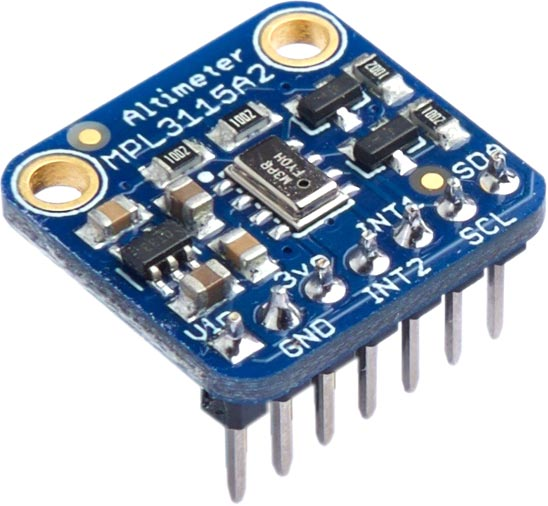## MPL3115A2 Altimeter

The MPL3115A2  from Freescale provides precision measurement of barometric pressure and altitude. It has a typical resolution of 1.5 Pascal resolution, and can resolve altitude to 0.3 meters. It has an I2C interface and includes a temperature sensor with ±1°C typical accuracy. It has an operating voltage range of 1.95 V to 3.6 V, so you'll need a regulator and logic-level conversion if you want to use it on a 5 V board.

Breakout boards are available from Adafruit  or SparkFun .The default I2C address is #x60 or 96.

#### Routines

The following routine go initiates a reading on one-shot mode. The parameters are as follows:

• alt: 0 = barometer mode, 1 = altimeter mode
• os: oversample ratio from 0 to 7
```(defun go (alt os)
; Enable data flags
(with-i2c (str 96)
(write-byte #x13 str) ; PT_DATA_CFG
(write-byte #x07 str))
(with-i2c (str 96)
(write-byte #x26 str) ; CTRL_REG_1
(write-byte (logior (ash alt 7) (ash os 3) 2) str))) ; Set OST bit```

The following integer routine rdy reads the temperature and pressure status flag and returns t if a reading is ready:

```(defun rdy ()
(with-i2c (str 96)
(write-byte #x00 str) ; STATUS
(restart-i2c str 1)

#### Temperature

The following integer routine tmp reads the temperature and returns a list of two integers, the whole number degrees, and the number of 1/16ths of a degree, in Celsius:

```(defun tmp ()
(go 0 0)
; Wait for PTDR bit set
(loop
(when (rdy) (return)))
(with-i2c (str 96)
(write-byte #x04 str) ; OUT_T_MSB
(restart-i2c str 2)
(list (- (logand msb #x7f) (logand msb #x80)) (ash lsb -4)))))```

For example:

```> (tmp)
(20 14)```

To convert this to a floating-point number of degrees on 32-bit versions of uLisp call temperature:

```(defun temperature ()
(let ((c (tmp)))
(+ (first c) (/ (second c) 16))))```

For example:

```> (temperature)
21.875```

#### Altitude

The following integer routine alt returns the altitude, in metres above sea level:

```(defun alt ()
(go 1 0)
; Wait for PTDR bit set
(loop
(when (rdy) (return)))
(with-i2c (str 96)
(write-byte #x01 str) ; OUT_P_MSB
(restart-i2c str 3)
(list (logior (ash msb 10) (ash csb 2) (ash lsb -6)) (logand (ash lsb -4) 3)))))```

It's returned as a list of two integers, in metres, and 1/4 metres.

To convert this to a floating-point number of metres on 32-bit versions of uLisp call altitude:

```(defun altitude ()
(let ((a (alt)))
(+ (first a) (/ (second a) 4))))```

#### Pressure

The following floating-point routine hpa returns the atmospheric pressure, in hPa:

```(defun hpa ()
(go 0 0)
; Wait for PTDR bit set
(loop
(when (rdy) (return)))
(with-i2c (str 96)
(write-byte #x01 str) ; OUT_P_MSB
(restart-i2c str 3)
```> (hpa)